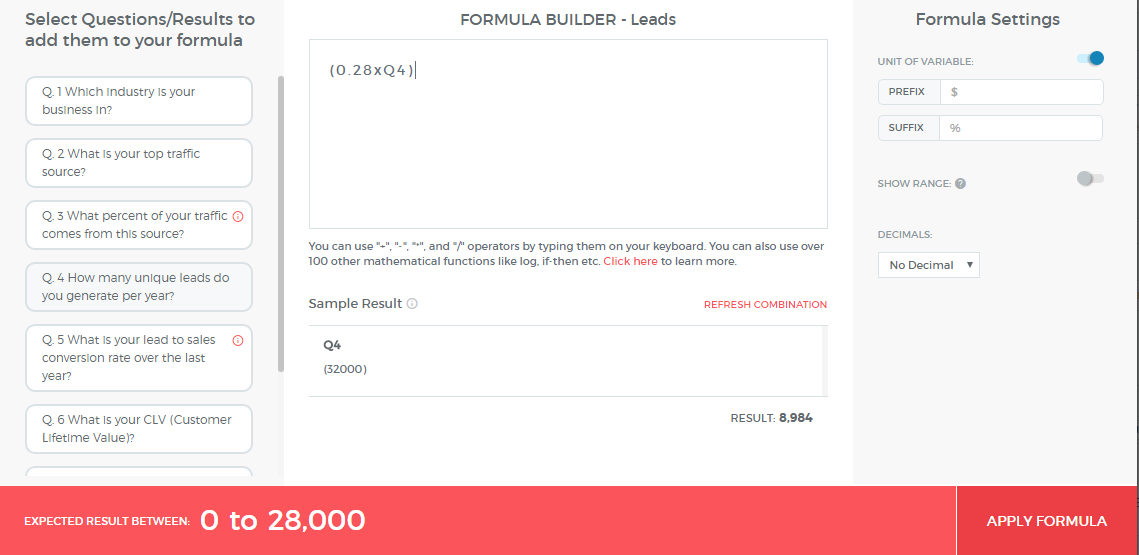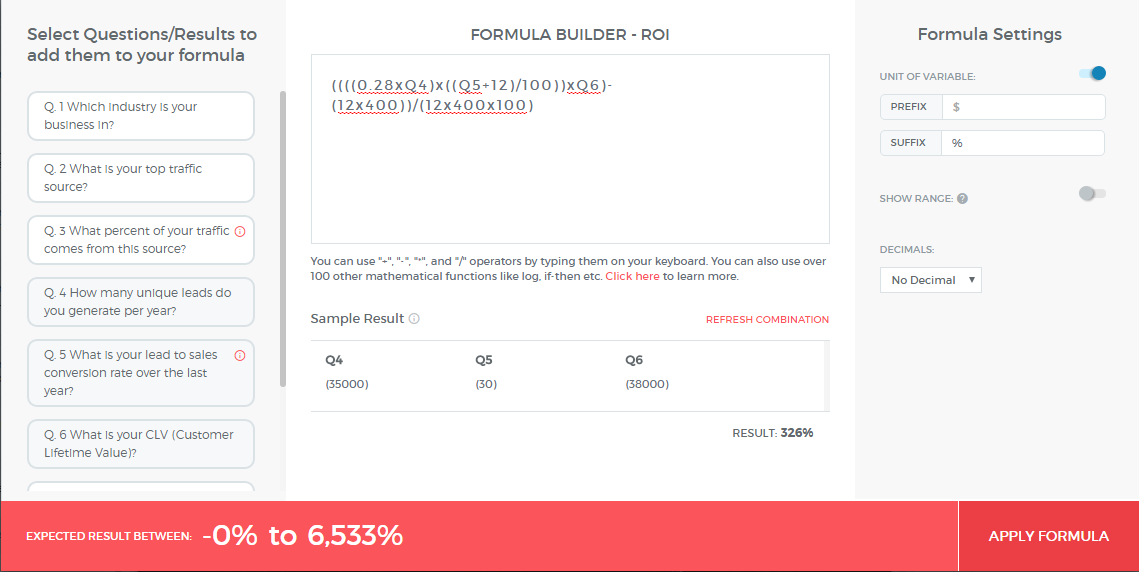# How to make an ROI Calculator?

Build an assessment using a calculator and a simple formula

# ROI Calculator

A RoI calculator usually requires a simple calculation along the lines of:

### 📘ROI Formula

RoI (%) = Net Gain (\$) / Total Cost of Investment (\$) * 100

Let’s break down this calculation with an example of calculating RoI of buying a subscription to the Outgrow Platform.

The first input we need is the Net Profit or simply put, how much money can one make net of costs. In formula terms:

### 📘Formula Breakdown

Net Gain (\$) = Marginal Gross Profit from Investment (\$) - Total Investment (\$)

While total investment is a an easy number to discern (since you are the seller you already know that answer), calculating Marginal Gross Profit can be slightly tricky and needs to be broken down further:

### 📘Formula Breakdown

Marginal Gross Profit (\$) = New Customers (#) x Marginal Gross Profit Per Customer (\$)

Note: Marginal Gross Profit per customer is sometimes referred to as Customer Lifetime value.

The customer will usually know the revenue per customer but might need help calculating the number of new customers he can get from calculators. That will not be obvious up front.

### 📘Formula Breakdown

New Customers (#) = Number of New Prospects x Sales Conversion Rate

For Outgrow, we know that our calculators increase conversions on landing pages by 28% on an average. Hence, the number of prospects or leads generated will be 28% more.

### 📘Formula Breakdown

New Customers From Calculators (#) = (Current Number of Prospects x 28%) x Sales Conversion RateThe current number of prospects and the sales conversion rates are both numbers that our users should know without any further breakdown. So our final formula break down will be:

### 📘Formula Breakdown

RoI (%) = Net Gain (\$) / Total Investment (\$) x 100

RoI (%) = (Marginal Gross Profit from Investment (\$) - Total Investment (\$)) / Total Investment (\$) x 100

RoI (%) = ((New Customers from Investment (#) x Marginal Gross Profit Per Customer (\$)) - Total Investment (\$)) / Total Investment (\$) x 100

RoI (%) = ((((28% of Current Number of Prospects) x Sales Conversion Rate) x Marginal Gross Profit Per Customer (\$)) - Total Investment (\$)) / Total Investment (\$) x 100So the entire calculator requires 3 simple inputs from the user and can calculate RoI.

Here, try this calculator in action.